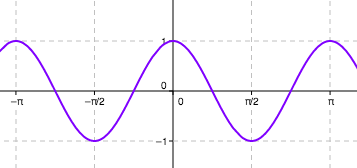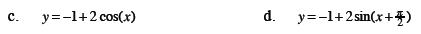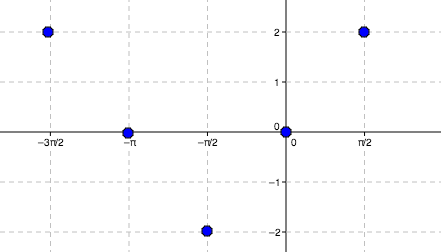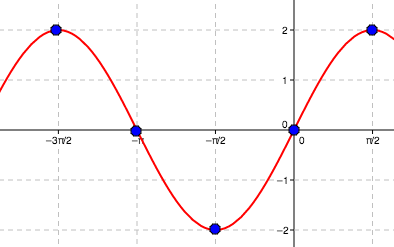Home > A2C > Chapter 11 > Lesson 11.3.4 > Problem11-170

11-170.
1. Graph at least one full cycle of each graph. Homework Help ✎

1. y = 2 sin x

2. y = cos (2x)

3. y = −1 + 2 cos(x)

4.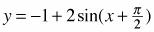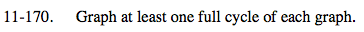For the following problems, use five points to sketch one cycle and then repeat the pattern.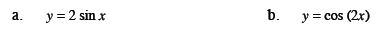Five points on the graph are displayed below.